## Section29.1Coulomb's Force

The French physicist Charles Coulomb studied electric force between charges extensively in 1780s using a torsion balance apparatus shown in Figure 29.1.1. Coulomb's torsion balance worked by charging two light-weight balls, one of which was fixed and the other attached to the end of a needle which was hung using a silver wire or hair to a torsion micrometer.

The torque on the balls was proportional to the angle of twist, which could be measured accurately. This allowed Coulomb to experiment the dependence of force on charge on each ball and on their separation.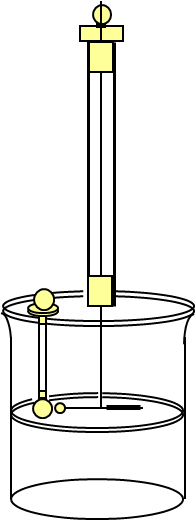Figure 29.1.1. Coulomb's torsion balance apparatus. A pith ball with charge is hung from a fine wire which can rotate when electric force from the charge on the fixed pith ball.

Coulomb found that electric force is proportional to the magnitudes of each charge, and inversely proportional to the square of the distance between them. To honor Coulomb for his contribution to our understanding of electricity, electric force is also called Coulomb force and the unit of charge is called Coulomb. In the following we will study this force mathematically.

It is interesting to note that Henry Cavendish also discovered the inverse square dependence of the electrostatic forces prior to Coulomb, but he did not publish his findings and his discovery was not known until James Clerk Maxwell publicized it in 1879. Other people who had known or suspected similarities with the Gravitational force included Benjamin Franklin and Joseph Priestly, whose work was the basis for Coulomb's experiments.

### Subsection29.1.1Coulomb's Law

Electric force $\vec F_e$ between two charges $q_1$ and $q_2$ separated by a distance $r$ has the following magnitude and direction.

\begin{align*} \amp \text{Magnitude:}\ \ F_e\propto \frac{|q_1| |q_2|}{r^2}. \\ \amp \text{Direction: use the rule: like charges repel, opposite charges attract.} \end{align*}

The direction of the force on any one of the charges depends on the type of the two charges and the charge on which the force acts as shown in Figure 29.1.2.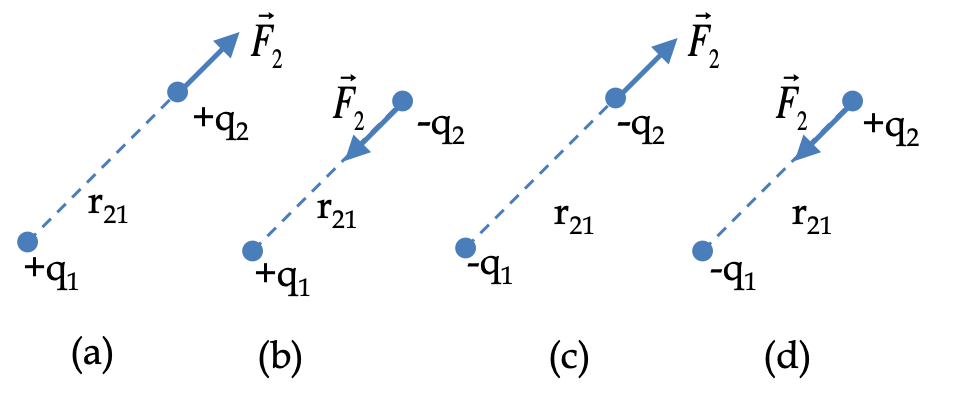Figure 29.1.2. Direction of Coulomb forces on a charge by another charge depends upon whether the two charges are of the same type or of different types. Coulomb force on charge $q_2$ by charge $q_1$ are shown for four different cases depending upon the type of charges $q_1$ and $q_2\text{.}$ Note the direction of the force depends on the relative type of each charge: (a) both positive, (b) $q_1$ positive, $q_2$ negative, (c) both negative and (d) $q_1$ negative, $q_2$ positive. For concreteness, this figure shows only force on $q_2\text{;}$ there is also an equal magnitude force in opposite direction on $q_1$ for each case.

Note that the magnitude of the force is always positive regardless of the charges involved. That is why I have placed an absolute sign $||$ around the symbols for charges. The magnitude of electric force $\vec F_e$ can be written as an equality by introducing a proportionality constant $k$ independent of $q_1\text{,}$ $q_2\text{,}$ or $r\text{.}$

\begin{equation} F_e = k \frac{|q_1| |q_2|}{r^2}.\tag{29.1.1} \end{equation}

The value of constant $k$ depends on the units in which $F_e\text{,}$ $q_1\text{,}$ $q_2\text{,}$ and $r$ are expressed. For instance, in the SI family of units, where force is expressed in Newton ($\text{N}$), charge in Coulomb ($\text{C}$) and distance in meter ($\text{m}$), the approximate value of $k$ is:

\begin{equation*} k \approx 9\times 10^9\frac{\text{N.m}^2}{\text{C}^2} \end{equation*}

Often one expresses $k$ in terms of another constant, denoted by symbol $\epsilon_0$ (read as epsilon zero), called the permittivity of vacuum or permittivity of free space by the following relation.

\begin{equation*} k \equiv \frac{1}{4\pi\epsilon_0}. \end{equation*}

The value of $\epsilon_0$ is then approximately given by

\begin{equation*} \epsilon_0 \approx 8.843 \times 10^{-12} \frac{\text{C}^2}{\text{N.m}^2}. \end{equation*}

There is another unit in common use, called the Gaussian-cgs system. In this unit $F$ is in units of $\text{dyne}\text{,}$ which is $10^{-5}\text{N}\text{,}$ $r$ in $\text{cm}\text{,}$ and $q$ in $\text{statcoulomb}\text{.}$ Then, $k = 1\text{,}$ and Coulomb's law takes a simple form.

\begin{equation*} F = \dfrac{q_1 q_1}{r^2},\ \ \text{(Gaussian-cgs units.)} \end{equation*}

For the sake of consistency with other introductory books, we will use the SI units only. You will find Gaussian-cgs units in older books and may be in some lab manuals.

Often, we need to work out components of Coulomb force from the magnitude and positions of the two charges. I will just write it here for your reference and to emphasize the vector aspect of the force. Let $F$ be the magnitude (positive), and $(x_1, y_1, z_1)$ and $(x_2, y_2, z_2)$ be the positions of charges $q_1$ and $q_2\text{,}$ respectively. The the Cartesian components of the force on $q_2$ will be

\begin{align} F_{\text{on 2},x} \amp = F\times \dfrac{x_2-x_1}{r_{12}}\times (\text{ sign of }q_1) \times (\text{ sign of }q_2),\tag{29.1.2}\\ F_{\text{on 2},y} \amp = F\times \dfrac{y_2-y_1}{r_{12}}\times (\text{ sign of }q_1) \times (\text{ sign of }q_2),\tag{29.1.3}\\ F_{\text{on 2},z} \amp = F\times \dfrac{z_2-z_1}{r_{12}}\times (\text{ sign of }q_1) \times (\text{ sign of }q_2),\tag{29.1.4} \end{align}

where $r_{12}$ is the direct distance between the two charges,

\begin{equation} r_{12} = \sqrt{ \left(x_1-x_2\right)^2 + \left(y_1-y_2\right)^2 +\left(z_1-z_2\right)^2 }.\tag{29.1.5} \end{equation}

### Subsection29.1.2Coulomb's Force in Vector Form

We can write Coulomb's law in a vector form by introducing a unit vector to represent the direction, and then multiplying the magnitude of the force by the unit vector.

We will like to write one formula that captures all four cases shown in Figure 29.1.2: both $q_1$ and $q_2$ positive, both $q_1$ and $q_2$ negative, $q_1$ positive but $q_2$ negative, and $q_1$ negative but $q_2$ positive. To illustrate the analytic way of writing these forces, I will illustrate here the force on $q_2$ which I will denote by $\vec F_\text{on 2}^\text{by 1}\text{,}$ or by $\vec F_2$ for brevity.

To do that we define a unit vector in the direction from $q_1$ to $q_2$ by $\hat u_{1\rightarrow 2}$ and the direct distance between the two charges by $r_{12}$ as in the figure to the right. With this notation, all four cases of force on charge $q_2$ can be stated by a single equation: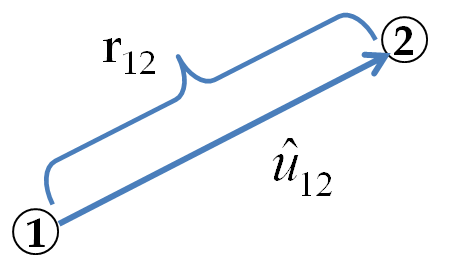The force on $q_2$ by $q_1$ be

\begin{equation} \vec F_\text{on 2}^\text{by 1} = \frac{1}{4\pi\epsilon_0}\frac{q_1 q_2}{r_{12}^2}\ \hat u_{1\rightarrow 2},\tag{29.1.6} \end{equation}

where we use a positive number for a positive charge and a negative number for a negative charge in order for the direction of the force to come out right. To write the force on $q_1$ by $q_2$ all you have to do in replace the unit vector by $\hat u_{2\rightarrow 1}\text{,}$ the vector from 2-to-1, which is negative of the unit vector $\hat u_{1\rightarrow 2}\text{,}$ i.e., $\hat u_{2\rightarrow 1} = - \hat u_{1\rightarrow 2}$

\begin{equation} \vec F_\text{on 1}^\text{by 2} = \frac{1}{4\pi\epsilon_0}\frac{q_1 q_2}{r_{12}^2}\hat u_{2\rightarrow 1} = - \frac{1}{4\pi\epsilon_0}\frac{q_1 q_2}{r_{12}^2}\hat u_{1\rightarrow 2}.\tag{29.1.7} \end{equation}

You can write unit vector $\hat u_{1\rightarrow 2}$ in terms of the Cartesian coordinate unit vectors $\hat u_x\text{,}$ $\hat u_y\text{,}$ and $\hat u_z\text{,}$ which you may have seen as $\hat i\text{,}$ $\hat j\text{,}$ and $\hat k\text{,}$ respectively. Let $\vec r_1$ and $\vec r_2$ be the position vectors of $q_1$ and $q_2$ respectively. Then, from the triangle of vectors in Figure 29.1.3, we can write the displacement vector from $q_1$ to $q_2\text{,}$ denoted by $\vec r_{12}$ in terms of the position vectors as

\begin{equation*} \vec r_{12} = \vec r_2 - \vec r_1. \end{equation*}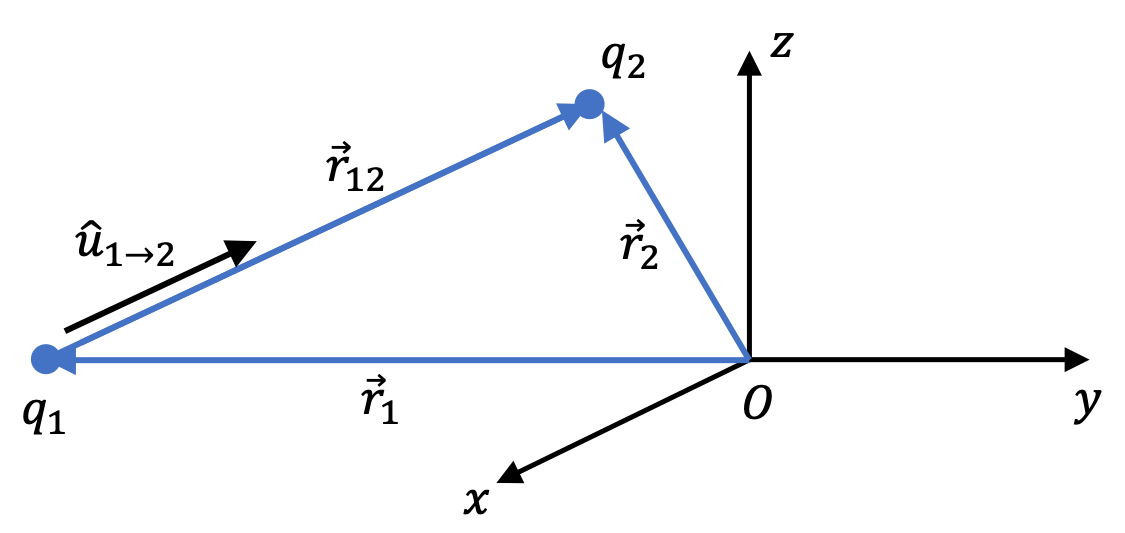Figure 29.1.3. Position, displacement, and unit vectors for Coulomb's law. Now, the position vectors can be simply written using the coordinates of the particles. This gives the following for the displacement vector.

\begin{equation*} \vec r_{12} = (x_2-x_1)\,\hat u_x + (y_2-y_1)\,\hat u_y + (z_2-z_1)\,\hat u_z. \end{equation*}

Unit vector in this direction, $\hat u_{1\rightarrow 2}\text{,}$ is just this vector divided by its magnitude.

\begin{equation} \hat u_{1\rightarrow 2} = \dfrac{ (x_2-x_1)\,\hat u_x + (y_2-y_1)\,\hat u_y + (z_2-z_1)\,\hat u_z}{ \sqrt{(x_2-x_1)^2 + (y_2-y_1)^2 + (z_2-z_1)^2 } }.\tag{29.1.8} \end{equation}

An electron an proton are separated by a distance of one Bohr radius, which is $5.3 \times 10^{−11}\text{ m}\text{.}$

(a) What is the magnitude of the electric force between them?

(b) What are the magnitudes of accelerations of electron and proton?

Data: $e = 1.60 \times 10^{-19}\text{ C}\text{,}$ $m_e = 9.11 \times 10^{-31}\text{ kg}\text{,}$ $m_p = 1.67 \times 10^{-27}\text{ kg}\text{.}$

Hint

(a) Strip the sign from the charge since magnitude. (b) Use $F/m\text{.}$

(a) $8.2 \times 10^{-8}\text{ N}\text{.}$ (b) $9.0 \times 10^{22}\text{ m/s}^2\text{,}$ $4.91 \times 10^{19}\text{ m/s}^2\text{.}$

Solution 1 (a)

We will use the formula for the magnitude of the Coulomb force. Note that we strip the sign when we just need the magnitude.

\begin{align*} F_e \amp = k \dfrac{q_1 q_2}{r^2}\\ \amp = 9 \times 10^9 \dfrac{1.60 \times 10^{-19} \times 1.60 \times 10^{-19}}{(5.3 \times 10^{−11})^2}\\ \amp = 8.2 \times 10^{-8}\text{ N}. \end{align*}
Solution 2 (b)

Dividing force by their masses will give us the magnitude of their accelerations.

\begin{align*} a_e \amp = \frac{8.2 \times 10^{-8}\text{ N}}{9.11 \times 10^{-31}\text{ kg}} = 9.0 \times 10^{22}\text{ m/s}^2.\\ a_p \amp = \frac{8.2 \times 10^{-8}\text{ N}}{1.67 \times 10^{-27}\text{ kg} } = 4.91 \times 10^{19}\text{ m/s}^2. \end{align*}

Two point particles with charges $+2\ \mu\text{C}$ and $+5\ \mu\text{C}$ are held in place by a $3\text{ N}$ forces on each charge pushing each towards the other. Find the distance between the charges.

Hint

Balance force on one of the charges and solve for $r\text{.}$

$17.3 \text{ cm}\text{.}$

Solution

Balancing the forces on one of the particles tells us that the magnitude of the electric force between the two charges is also $3\text{ N}\text{.}$ Let us denote by $F\text{.}$

\begin{equation*} k\frac{|q_1| |q_2|}{r^2} = F. \end{equation*}

Solving for $r^2$ we get

\begin{equation*} r^2 = k\frac{|q_1| |q_2|}{F}. \end{equation*}

Putting in the numbers we get

\begin{align*} r^2 \amp = 9\times 10^9 \ \text{N.m}^2/\text{C}^2 \times \frac{2\times 10^{-6}\ \text{C} \times 5\times 10^{-6}\ \text{C} }{3\ \text{N}}\\ \amp = 0.03 \ \text{m}^2. \end{align*}

This gives $r = 0.173\text{ m} = 17.3 \text{ cm}\text{.}$

Two charges of magnitudes $q_1 = + 5\ \mu\text{C}$ and $q_2 = + 8\ \mu\text{C}$ are kept separated by a distance of $25\text{ cm}\text{.}$ When a third charge $q_3= +10\ \mu\text{C}$ is brought at a particular point on the line joining the first two charges, the third charge does not experience any force on it.

(a) Find the distance from $q_1$ of this special point between the two charges.

(b) Will the location of these special points change if we double $q_3$ to $+20\ \mu\text{C}\text{?}$

(c) What are the magnitudes of forces $F_1$ and $F_2$ when $q_3 = +10\ \mu\text{C}$ is at that special location between them?

Hint

(a) Balance two forces on $q_3\text{.}$ (b) Think, what happened in your calculation? (c) Balance forces on $q_1$ to get $F_1\text{.}$

(a) $11\text{ cm}\text{,}$ (b) No, (c) $43.0\text{ N},\ 42.5\text{ N}\text{.}$

Solution 1 (a)

(a) Clearly, the forces on $q_3$ will be balanced at the special point. Let that point be $x$ away from $q_1$ towards $q_2\text{.}$ Balancing the force on $q_3$ we get the following equation.

\begin{equation*} k \dfrac{ q_1 \, q_3 }{ x^2} = k \dfrac{ q_2 \, q_3 }{ (0.25 - x)^2}. \end{equation*}

Canceling the common factors and rearranging terms we get

\begin{equation*} 5(0.25 - x)^2 = 8x^2. \end{equation*}

Expanding and rearranging we get

\begin{equation*} 3 x^2 + 2.5 x - 0.3125 = 0. \end{equation*}

Solving this equation gives us two values of $x\text{.}$

\begin{equation*} x = \dfrac{1}{12}\left(-5 \pm 2\sqrt{10} \right) = -0.944,\ 0.11. \end{equation*}

The first root is not a point between charges $q_1$ and $q_2\text{.}$ therefore, we will just reject it and use the second root. That is, the special point is $11\text{ cm}$ from $q_1\text{.}$

Solution 2 (b)

(b) No, since $q_3$ cancels out, the cancellation will occur at the special point for all values of $q_3\text{.}$

Solution 3 (c)

(c) We note that at the instant in question, force $F_1$ must balance the net electric forces on $q_1$ by $q_2$ and $q_3\text{.}$ This will give

\begin{align*} F_1 \amp = k |q_1| \left[ \frac{|q_2|}{r_{12}^2} + \frac{|q_3|}{r_{13}^2} \right]\\ \amp = 9\times 10^9 \times 5\times 10^{-6} \left[ \frac{8\times 10^{-6}}{0.25^2} + \frac{10\times 10^{-6}}{0.11^2} \right] = 43.0\text{ N}. \end{align*}

Similarly, we get

\begin{align*} F_2 \amp = k |q_2| \left[ \frac{|q_1|}{r_{21}^2} + \frac{|q_3|}{r_{23}^2} \right]\\ \amp = 9\times 10^9 \times 8\times 10^{-6} \left[ \frac{5\times 10^{-6}}{0.25^2} + \frac{10\times 10^{-6}}{(0.25-0.11)^2} \right] = 42.5\text{ N}. \end{align*}

Two Styrofoam balls of equal diameter are painted with a silver paint. An unknown amount of charge is put on one of them and then the other ball is brought in contact with it. As a result, an equal amount of charge is distributed on each ball.

When the balls are hung by using a 1-meter long cotton thread each from the same point, they repel each other and come to rest with 60 degrees angle between them. If the masses of the balls are 10 grams each, what is the amount of charge on each ball?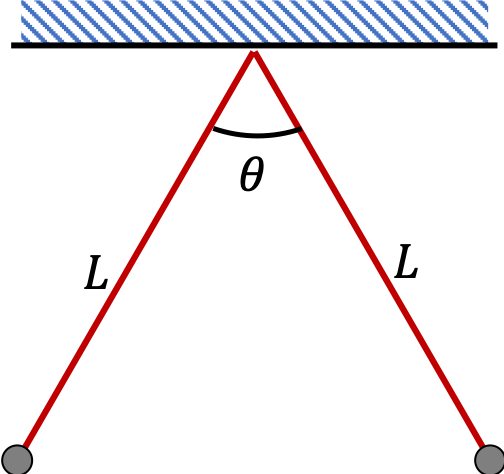Hint

Balance the forces on one of the charges. It will be a two-dimensional case. So, use components when working with vector equations.

Either $+2.5\ \mu\text{C}$ or $-2.5\ \mu\text{C}$

Solution

Since the acceleration of each ball is zero, net force on each ball would also be zero. To obtain relations among the forces we will identify all the forces on one of the balls, say #2 in Figure 29.1.10, and then set their vector sum to zero.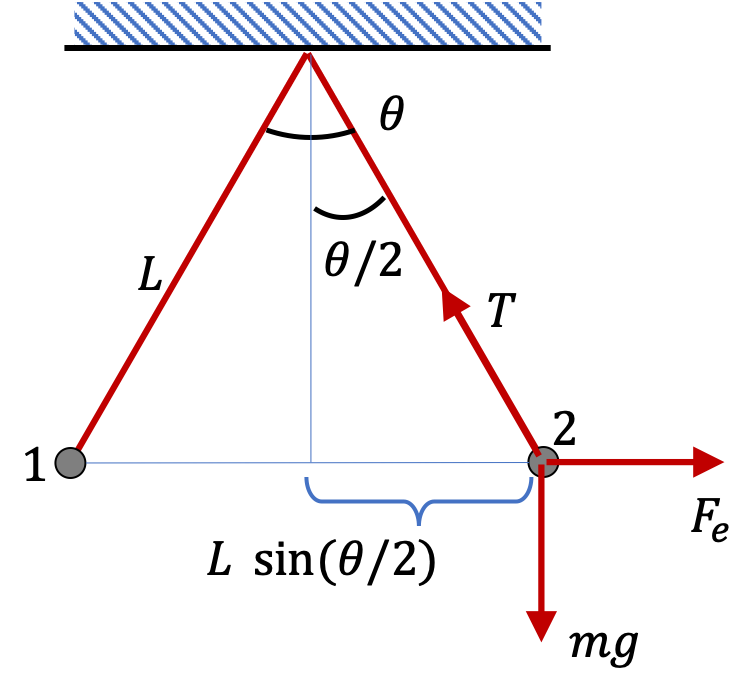Figure 29.1.10. Forces on one of the charged balls are tension $T\text{,}$ weight $W=mg\text{,}$ and Coulomb $F_e\text{.}$

Let $F_e\text{,}$ $T\text{,}$ and $W$ denote the magnitudes of the Coulomb force, the tension in the string, and the weight of the ball, respectively. Then, from the free-body diagram shown in Figure 29.1.10, we obtain the following components of the equation $\vec F_\text{net}=0\text{.}$

Balancing the horizontal and vertical components of forces gives the following two equation.

\begin{align*} \amp F_e - T\, \sin(\theta/2) = 0 \\ \amp T\, \cos(\theta/2) - W = 0 \end{align*}

Therefore, the Coulomb force must have the following magnitude.

\begin{equation*} F_e = W\,\tan(\theta/2). \end{equation*}

This must equal the magnitude of this force from Coulomb's law. Since the balls have the same amount of charge, say $q\text{,}$ we get

\begin{equation*} k \dfrac{q^2}{\left(2 L\,\sin(\theta/2) \right)^2 } = W\,\tan(\theta/2). \end{equation*}

Solving for $q$ we have

\begin{equation*} q = \pm \left(2 L \sin(\theta/2) \right)\sqrt{(W/k)\tan(\theta/2)}. \end{equation*}

Now, we put in the numbers $L=1\text{ m}\text{,}$ $\theta = 60^{\circ}\text{,}$ $W = 0.01\times9.8\text{ N}\text{,}$ $k = 9.0 \times 10^9 \text{N.m}^2/\text{C}^2$ to obtain the numerical value of the charge $q\text{.}$

\begin{equation*} q = \pm 2.5 \times 10^{-6}\ \text{C} = \pm 2.5\ \mu\text{C}. \end{equation*}

Since the two balls repel each other, the charges on the two are of the same type, either both are positive or both negative. They are both either $+2.5\ \mu\text{C}$ or $-2.5\ \mu\text{C}\text{.}$ However, based on the data given, we cannot determine which is the case here. If we knew the type for one of the charges, we would have immediately known the type for the other.

Two copper spheres of radius $2\text{ cm}$ are held $1\text{ m}$ apart. If proton's charge were to be $1\%$ greater than an electron's charge in magnitude, what will be the magnitude of force between the two spheres? Cu: density = $8.92\text{ g/cc}\text{,}$ atomic number = $29\text{,}$ atomic weight = $63.54\text{.}$

Hint

Each sphere will have net positive charge.

$1.5\times 10^{20}\ \textrm{N}\text{.}$

Solution

Let $N$ denote the number of atoms in one sphere. Then each sphere has $29\times N$ electrons and $29 \times N$ protons. With $-e$ denoting the charge on one electron we find that the net charge on each sphere will be

\begin{equation*} q = 0.01 \times 29\times N \times e. \end{equation*}

With $r = 1$ m the force between the spheres will be

\begin{equation*} F_e = k \frac{q^2}{r^2}. \end{equation*}

Now we work out the numerical values. First we find the value of $N\text{.}$ We recall from Chemistry that there are an Avogadro number $N_A =6.022 \times 10^{23}$ atoms in one mole and that the atomic weight $M_A$ refers to the mass of one mole in grams. Therefore, if we know the mass $M$ of the sphere we will be able to find the number of atoms. The mass can be computed from the volume $V$ and density $\rho\text{.}$

\begin{equation*} M = \rho V =\rho \times \frac{4}{3} \pi R^3, \end{equation*}

where $R$ is the radius of the ball. Therefore $N$ is

\begin{equation*} N = \frac{M}{M_A} \times N_A, \end{equation*}

where both $M$ and $M_A$ are in grams. Putting these together we find the magnitude of the electric force between the two spheres to be

\begin{align*} F_e \amp = k \frac{q^2}{r^2} = k \frac{( 0.01 \times 29\times N \times e)^2}{r^2} \\ \amp = k \frac{( 0.01 \times 29 \times e)^2}{r^2}\times \left(\frac{M(\textrm{in gram})}{M_A} \times N_A \right)^2. \end{align*}

Now putting the numerical values gives $1.5\times 10^{20}\ \textrm{N}\text{.}$